# IMAGE SEGMENTATION USING PCA BY VASUDHA ANUGONDA Image

• Slides: 35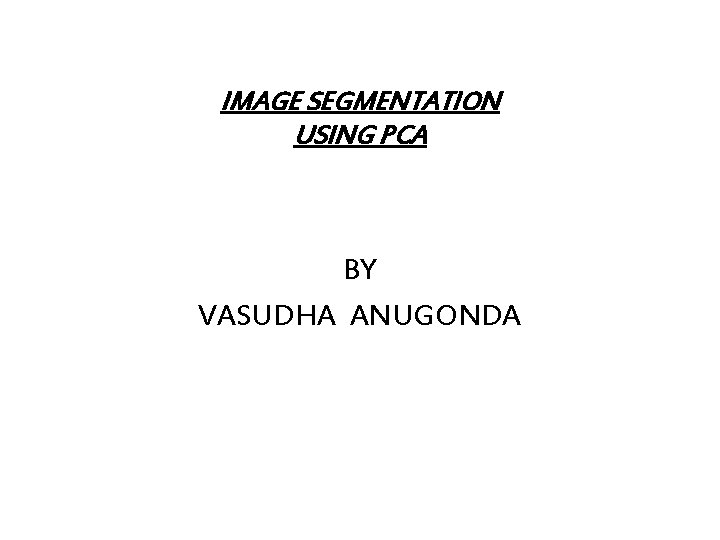IMAGE SEGMENTATION USING PCA BY VASUDHA ANUGONDAImage Segmentation • To distinguish objects in an image • Two types 1) Edge based 2) Region based We are using region based segmentation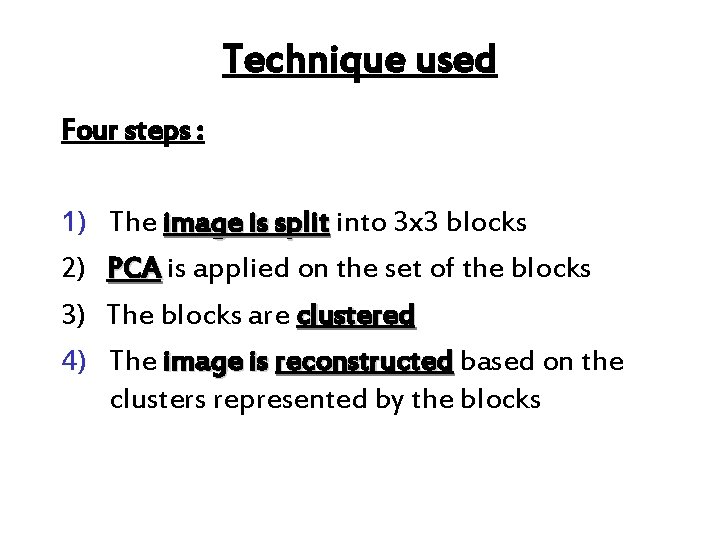Technique used Four steps : 1) 2) 3) 4) The image is split into 3 x 3 blocks PCA is applied on the set of the blocks The blocks are clustered The image is reconstructed based on the clusters represented by the blocks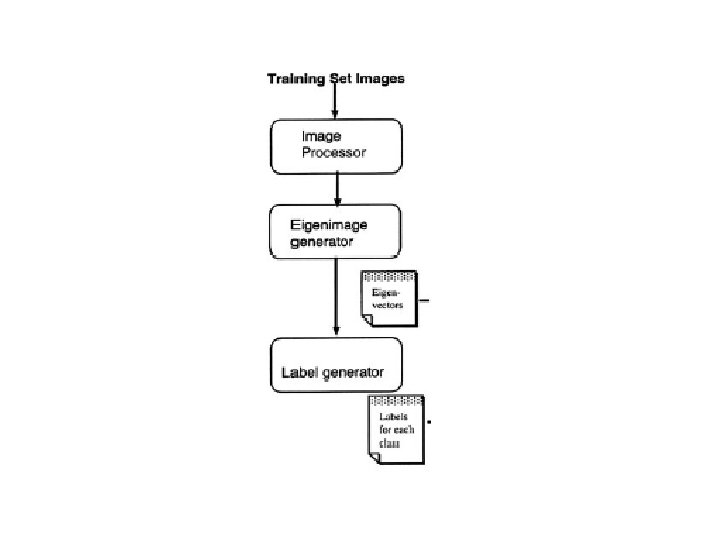Step 1 : • image is split into sliding blocks of 3 x 3 such that each pixel is represented by its 8 neighborhood.Step 2 : PRINCIPAL COMPONENT ANALYSIS: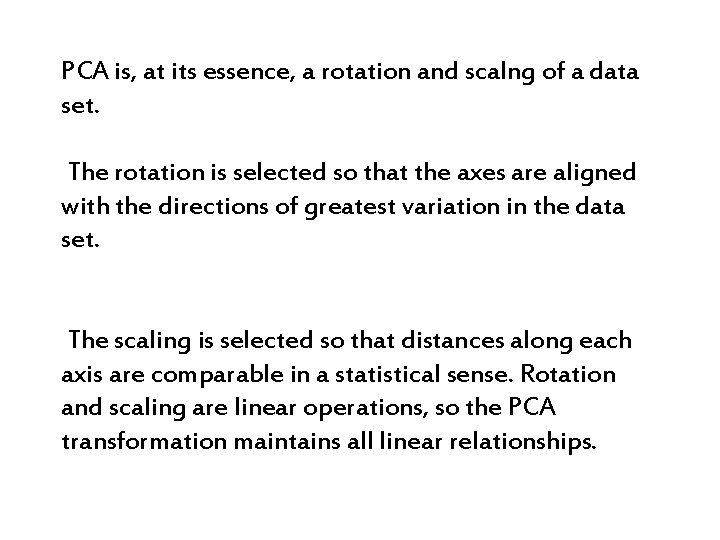PCA is, at its essence, a rotation and scalng of a data set. The rotation is selected so that the axes are aligned with the directions of greatest variation in the data set. The scaling is selected so that distances along each axis are comparable in a statistical sense. Rotation and scaling are linear operations, so the PCA transformation maintains all linear relationships.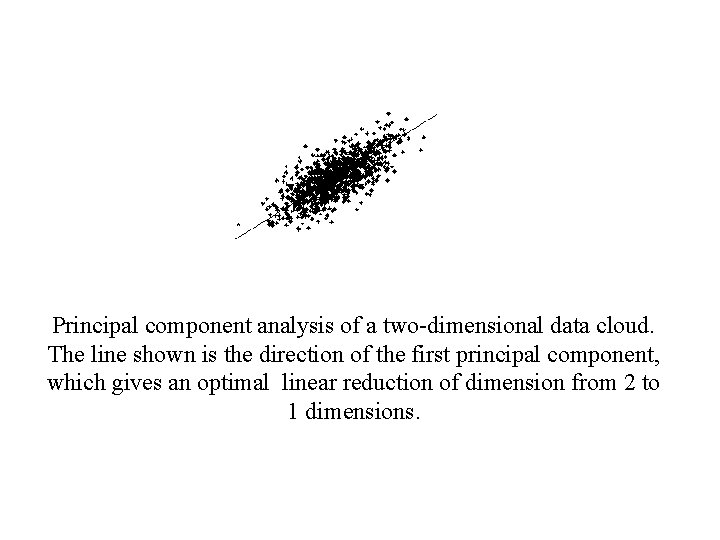Principal component analysis of a two-dimensional data cloud. The line shown is the direction of the first principal component, which gives an optimal linear reduction of dimension from 2 to 1 dimensions.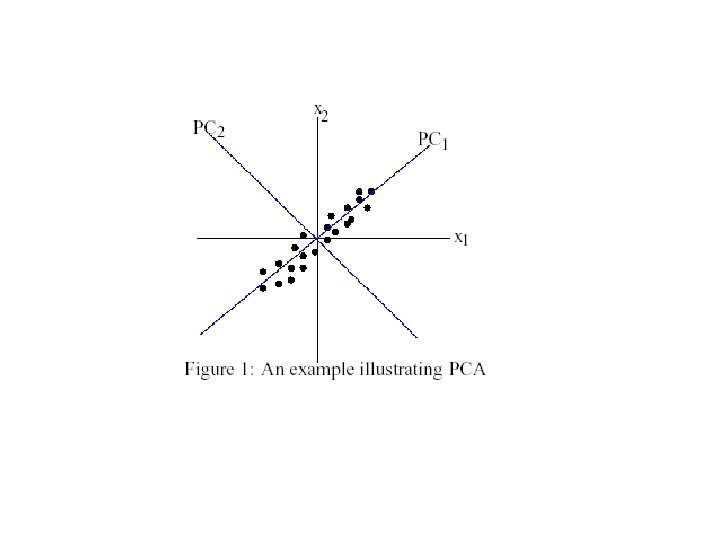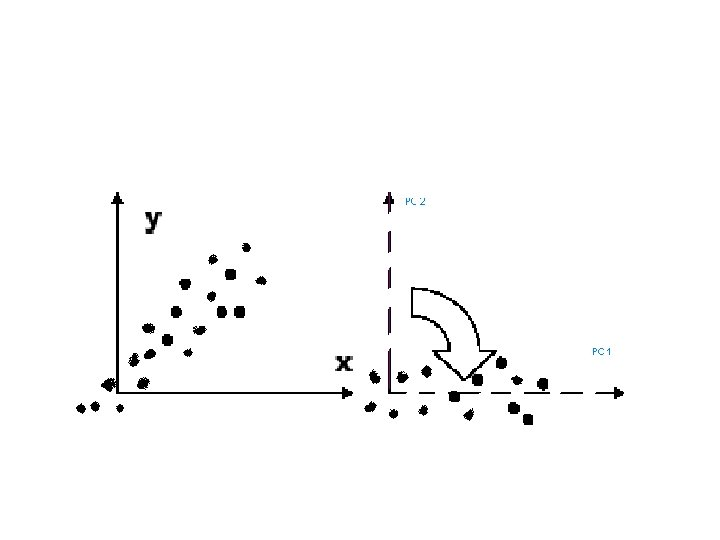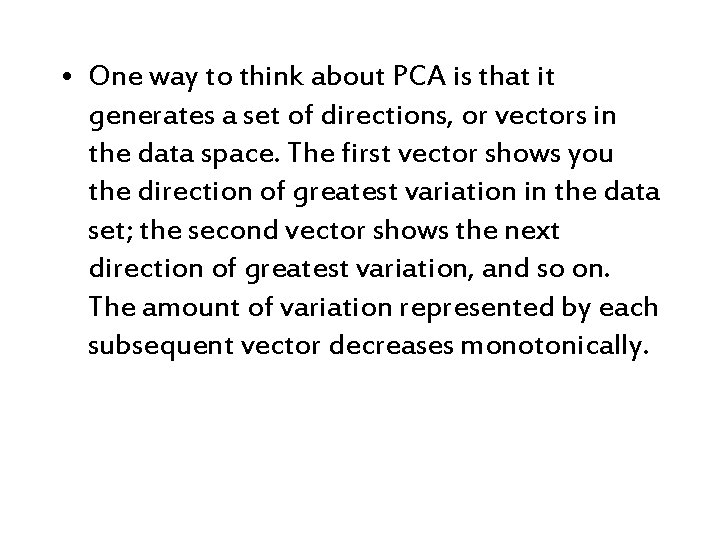• One way to think about PCA is that it generates a set of directions, or vectors in the data space. The first vector shows you the direction of greatest variation in the data set; the second vector shows the next direction of greatest variation, and so on. The amount of variation represented by each subsequent vector decreases monotonically.BACKGROUND MATHEMATICS • The sub images are stored as stack one behind the other. • The relation among all the pixels of the blocks are calculated • It is stored in covariance matrix • The eigen vectors of the covariance matrix are found.• The resulting matrix T is already the transform that concentrates the energy of an average n * n texture block in the best possible manner. Each texture block in the original image can now be transformed using that transform.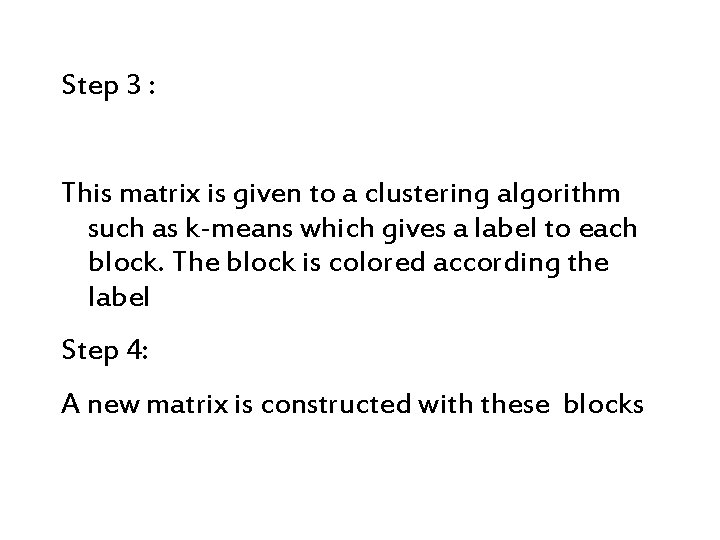Step 3 : This matrix is given to a clustering algorithm such as k-means which gives a label to each block. The block is colored according the label Step 4: A new matrix is constructed with these blocks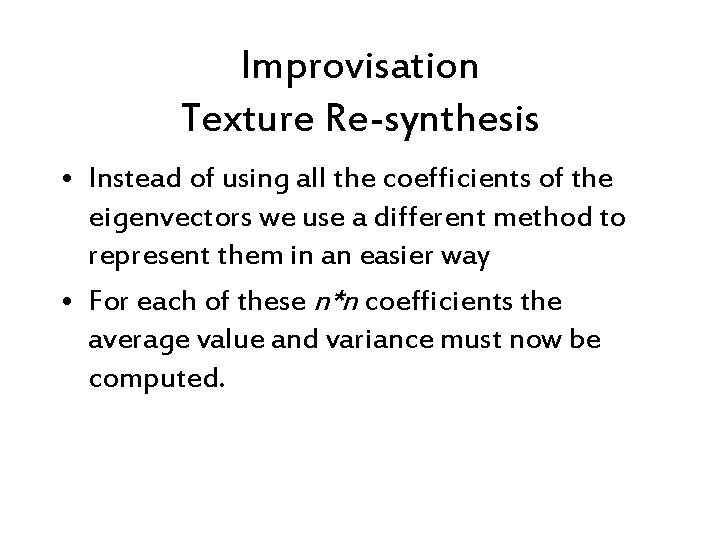Improvisation Texture Re-synthesis • Instead of using all the coefficients of the eigenvectors we use a different method to represent them in an easier way • For each of these n*n coefficients the average value and variance must now be computed.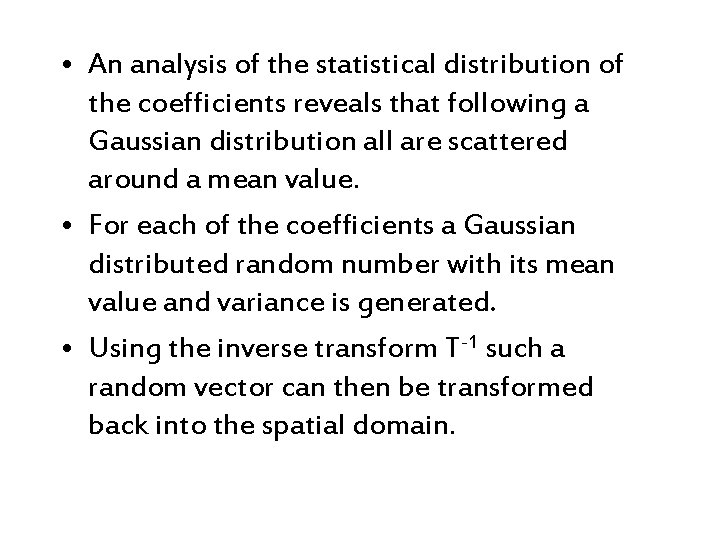• An analysis of the statistical distribution of the coefficients reveals that following a Gaussian distribution all are scattered around a mean value. • For each of the coefficients a Gaussian distributed random number with its mean value and variance is generated. • Using the inverse transform T-1 such a random vector can then be transformed back into the spatial domain.Original image which is segmented using PCA image which is segmented after resynthesis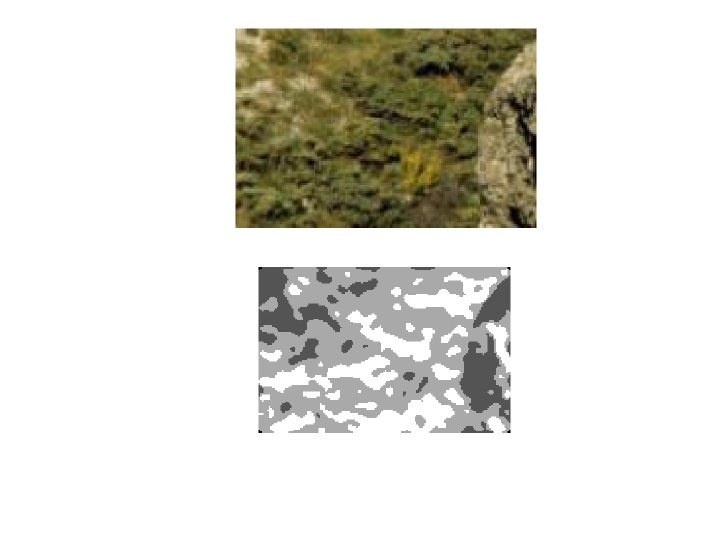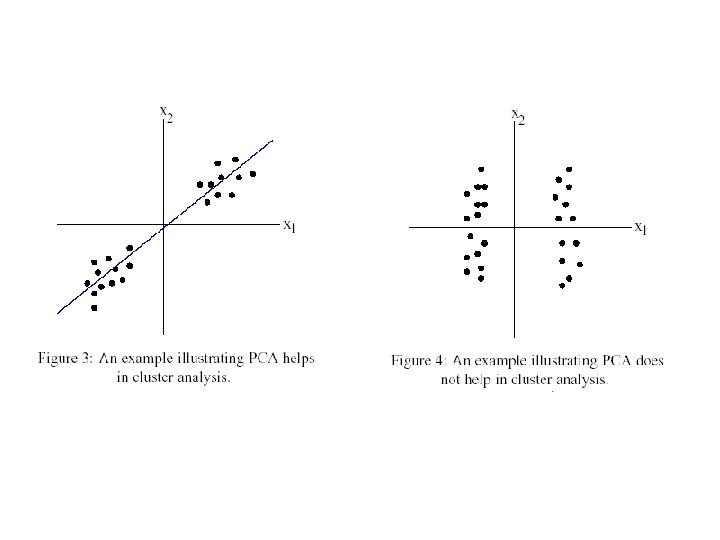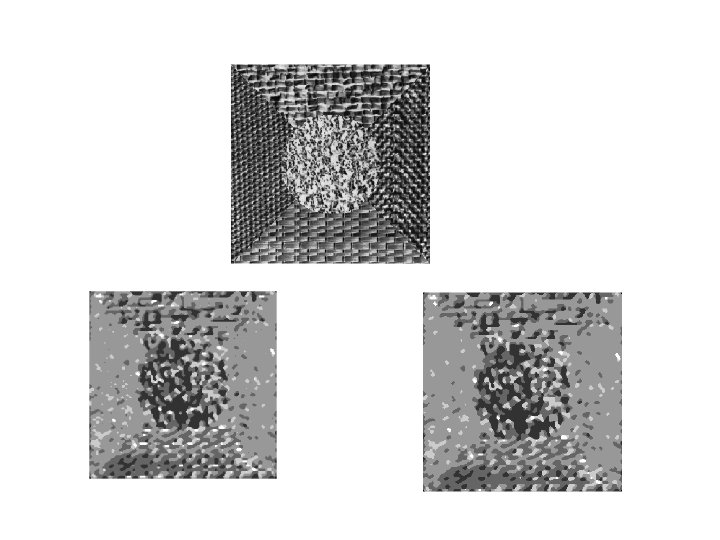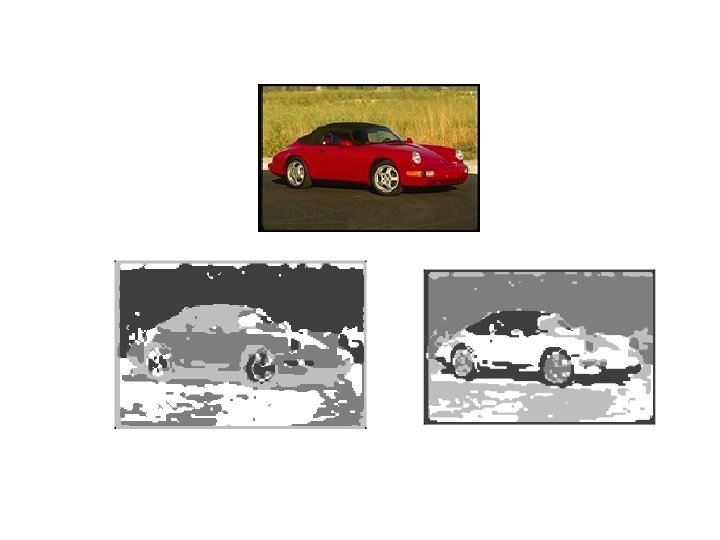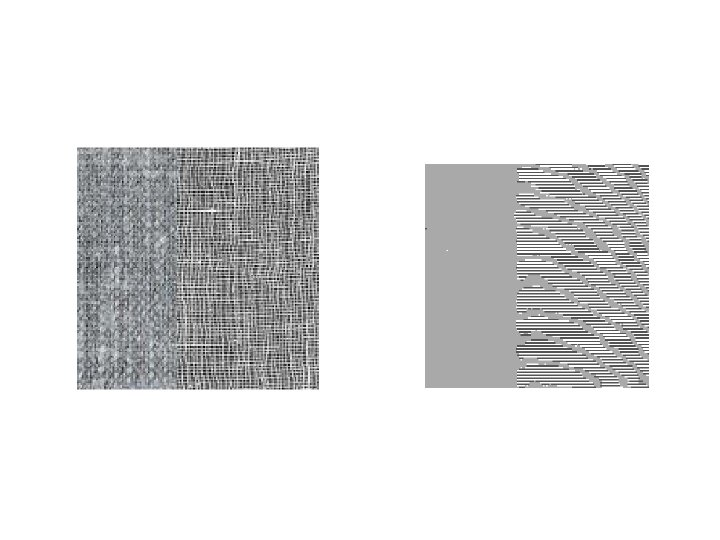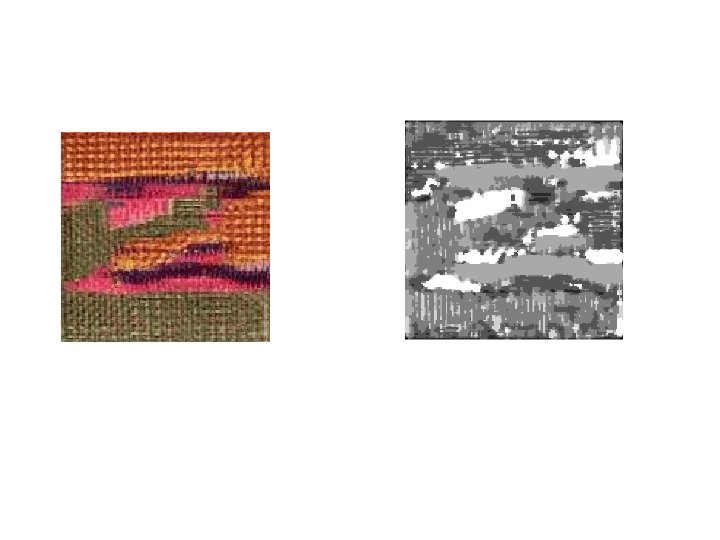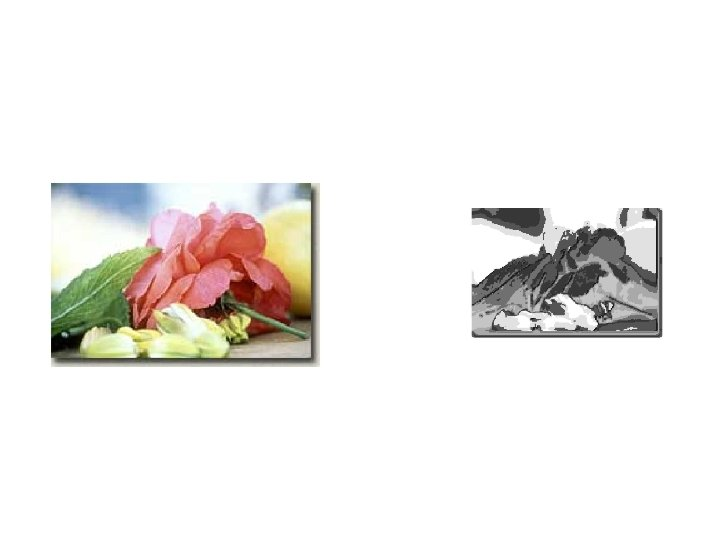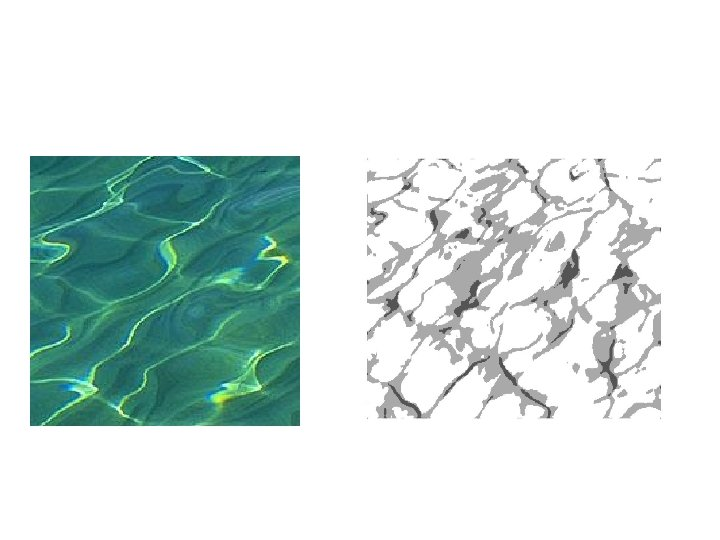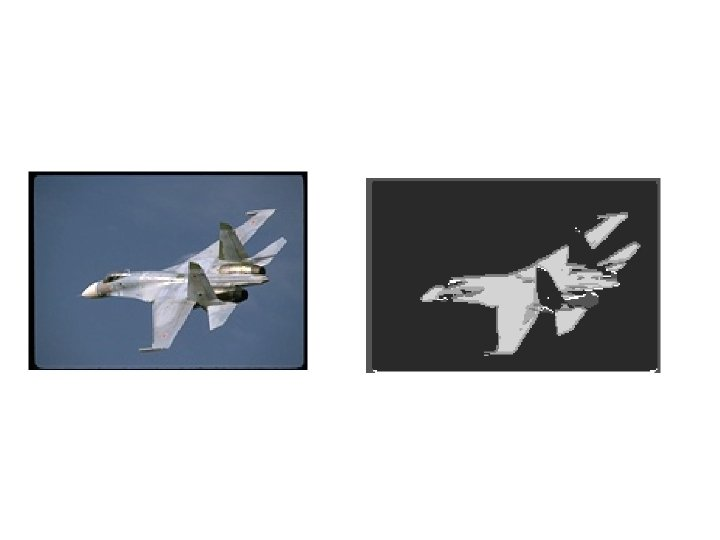• PCA fails sometimes because of the texture similarity , but difference in color values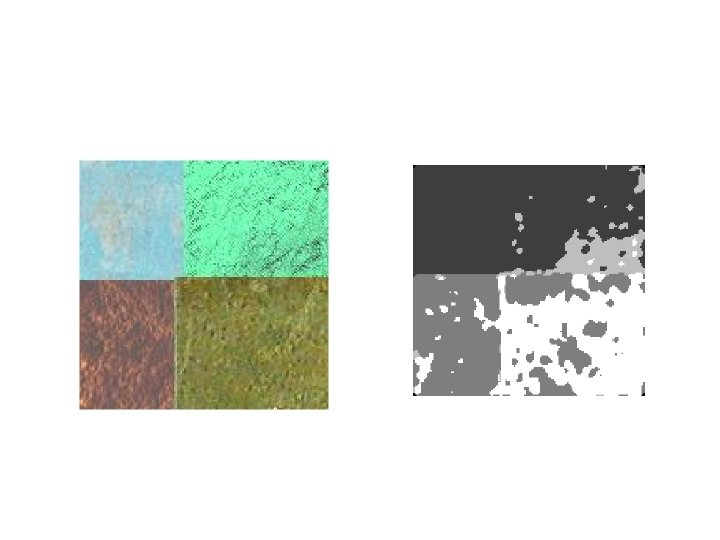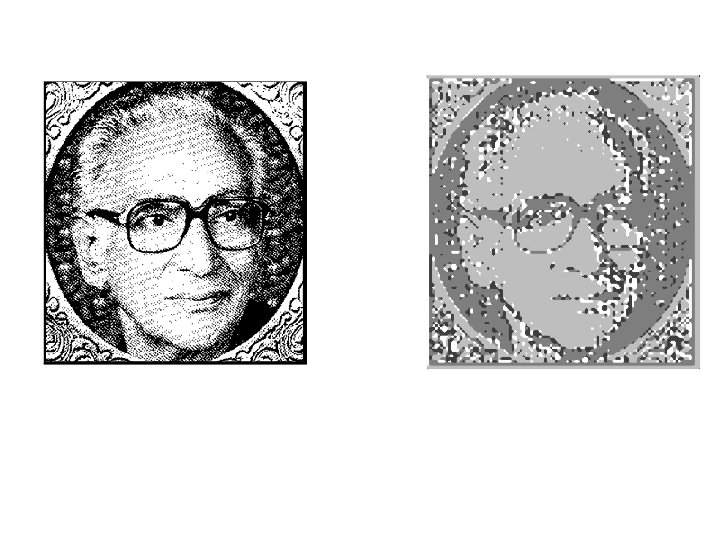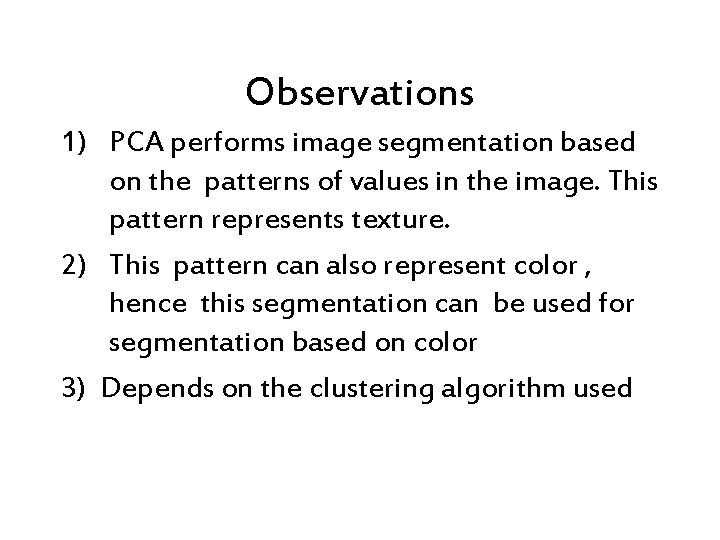Observations 1) PCA performs image segmentation based on the patterns of values in the image. This pattern represents texture. 2) This pattern can also represent color , hence this segmentation can be used for segmentation based on color 3) Depends on the clustering algorithm used4)The re-synthesis of textures using gaussian distribution can be used as an image enhancement technique. 5) Using texture re-synthesis and PCA together produces a good segmented image. 6) Same computation required. 7)This can also be used to transmit images in a compact form.Applications 1) 2) 3) 4) 5) Image segmentation Object detection Image enhancement Image compression Reading of satellite and radar imagesReferences • http: //faculty. washington. edu/kayee/pca/p ca. pdf • http: //www. informatik. unimannheim. de/pi 4/publications/library/Hae nselmann 2002 a. pdf • http: //www. cs. otago. ac. nz/cosc 453/student _tutorials/principal_components. pdf